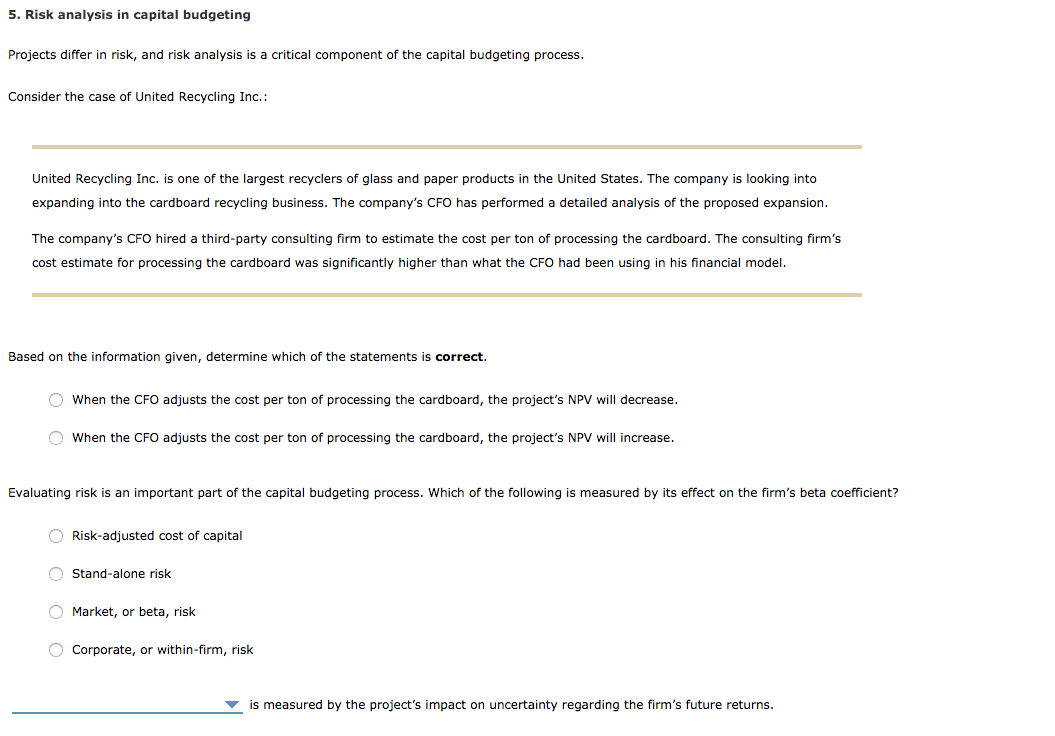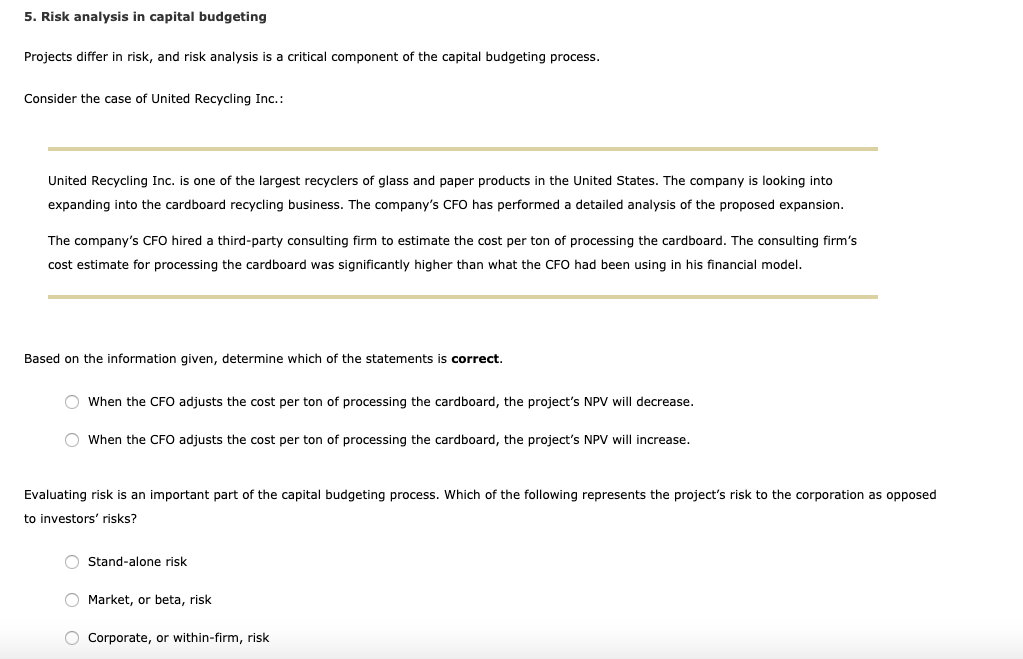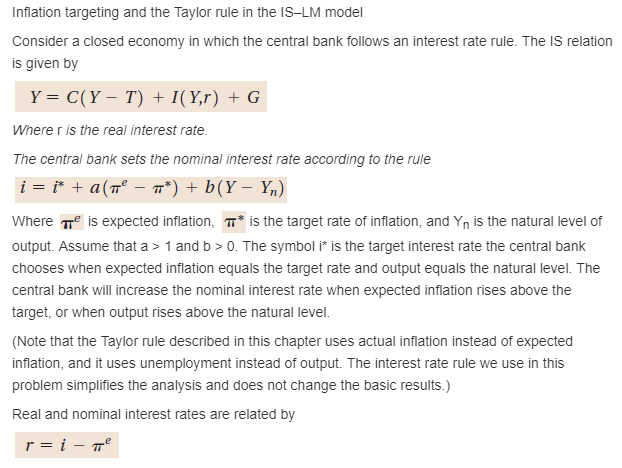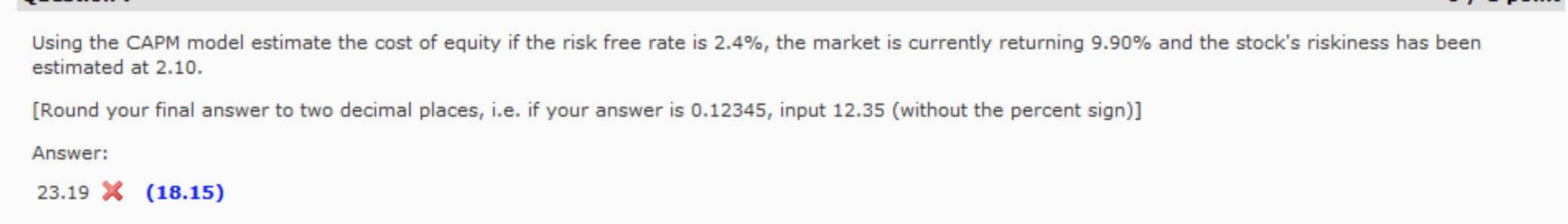# When treating futures using the cost of carry model, where does inflation risk be considered? Provide analysis.

When treating futures using the cost of carry model, where does inflation risk be considered? Provide analysis.

Many factors affect futures prices, such as interest rates, storage costs and dividend income.

The futures price of non-dividend and non-storable assets is a function of the risk-free interest rate, the spot price and the expiry time.

Assets expected to pay income will lower futures prices.

Since the seller of the futures incorporates the cost into the contract, storage costs will always increase the futures price.

The convenience rate of return (indicating the gains of owning another asset rather than owning futures) lowers the price of futures.

##### Add Answer of: When treating futures using the cost of carry model, where does inflation risk be considered? Provide analysis.
Similar Homework Help Questions
• ### 1. What is the cost-of-carry model for pricing futures and forward contracts? Provide a “fair” futures...

1. What is the cost-of-carry model for pricing futures and forward contracts? Provide a “fair” futures price for each of the following assets: a. S&P 500 Index: Contract June (3 months away); Current value of S&P 500 2450; 3-month Libor=2%.

• ### What specific economic factors need to be considered when conducting economic analysis? Provide j...

What specific economic factors need to be considered when conducting economic analysis? Provide justification to your answer and include three specific examples.

• ### 5. Risk analysis in capital budgeting Projects differ in risk, and risk analysis is a critical...5. Risk analysis in capital budgeting Projects differ in risk, and risk analysis is a critical component of the capital budgeting process. Consider the case of United Recycling Inc.: United Recycling Inc. is one of the largest recyclers of glass and paper products in the United States. The company is looking into expanding into the cardboard recycling business. The company's CFO has performed a detailed analysis of the proposed expansion. The company's CFO hired a third-party consulting firm to estimate...

• ### 5. Risk analysis in capital budgeting Projects differ in risk, and risk analysis is a critical...5. Risk analysis in capital budgeting Projects differ in risk, and risk analysis is a critical component of the capital budgeting process. Consider the case of United Recycling Inc.: United Recycling Inc. is one of the largest recyclers of glass and paper products in the United States. The company is looking into expanding into the cardboard recycling business. The company's CFO has performed a detailed analysis of the proposed expansion. The company's CFO hired a third-party consulting firm to estimate...

• ### 6. 7. Inflation targeting and the Taylor rule in the IS-LM model Consider a closed economy...6. 7. Inflation targeting and the Taylor rule in the IS-LM model Consider a closed economy in which the central bank follows an interest rate rule. The IS relation is given by Y C(Y- T) I(Y,r) G Where r is the real interest rate. The central bank sets the nominal interest rate according to the rule i = i* + a(n° =- T*) + b(Y- Y1) Where T is expected inflation, T* is the target rate of inflation, and Yn...

• ### 6. 7. Inflation targeting and the Taylor rule in the IS-LM model Consider a closed economy...6. 7. Inflation targeting and the Taylor rule in the IS-LM model Consider a closed economy in which the central bank follows an interest rate rule. The IS relation is given by Y C(Y- T) I(Y,r) G Where r is the real interest rate. The central bank sets the nominal interest rate according to the rule i = i* + a(n° =- T*) + b(Y- Y1) Where T is expected inflation, T* is the target rate of inflation, and Yn...

• ### please answer Question 7: Inflation targeting and the Taylor rule in the IS-LM model Consider a...please answer Question 7: Inflation targeting and the Taylor rule in the IS-LM model Consider a closed economy in which the central bank follows an interest rate rule. The IS relation is given by Y C(Y- T) I(Y,r) G Where r is the real interest rate. The central bank sets the nominal interest rate according to the rule i = i* + a(n° =- T*) + b(Y- Y1) Where T is expected inflation, T* is the target rate of inflation,...

• ### Using the eight factor model of HCS does the United States provide true access to healthcare?

Using the eight factor model of HCS does the United States provide true access to healthcare?

• ### Using the CAPM model estimate the cost of equity if the risk free rate is 2.4%,...Using the CAPM model estimate the cost of equity if the risk free rate is 2.4%, the market is currently returning 9.90% and the stock's riskiness has been estimated at 2.10. [Round your final answer to two decimal places, i.e. if your answer is 0.12345, input 12.35 (without the percent sign)] Answer: 23.19 X (18.15)

• ### Which one of the following factors is not considered in calculating the firm’s cost of equity? risk free rate of retu...

Which one of the following factors is not considered in calculating the firm’s cost of equity? risk free rate of return beta interest rate on corporate debt expected return on equities difference between expected return on stocks and the risk free rate of return Which one of the following factors is not considered in calculating the firm’s cost of capital? cost of equity interest rate on debt the firm’s marginal tax rate book value of debt and equity the firm’s...

Free Homework App